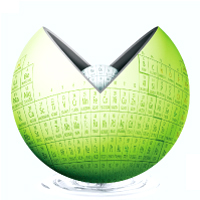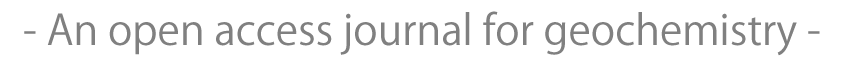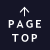JavaScript requeired.Published for geochemistry community from Geochemical Society of Japan.

## Determination of activation energy and pre-exponential factor for individual compounds on release from kerogen by a laboratory heating experiment

Masahiro Oba, Hajime Mita, Akira Shimoyama
Geochemical Journal, Vol. 36, No. 1, P. 51-60, 2002

#### ABSTRACT

Kerogen recovered from Neogene Shinjo sediments was heated from room temperature to 800°C using a thermogravimetric analyzer (TG). Organic compounds released from kerogen during heating were trapped and analyzed by a gas chromatograph (GC)-mass spectrometer (MS). Among these compounds, benzene, hexane, toluene, phenol, heptane, and indene were selected to determine temperatures at which maximum release of these compounds occurred based on TG-MS peak profiles. This analysis was carried out using programmed heating rates (Hr) of 5, 10, 20, 30, and 60°C min-1 and five temperatures at peak tops (Tmax in absolute temperature) were determined for each compound. The plot of 1/Tmax vs. ln(Hr/(Tmax)2) for each compound showed a good straight line with a high correlation coefficiency. Accordingly, a mean activation energy (E0) and pre-exponential factor (A) at E0 for each compound on the release from kerogen were calculated, using the equation of the approximate method (or Gaussian E-distribution method) by Braun and Burnham (1987), ln(Hr/(Tmax)2) = −E0/RTmax + ln(AR/E0) where R is the ideal gas constant. The activation energies and pre-exponential factors of these compounds were in a range of 43.1 to 60.9 kcal/mol and of 3.56 × 1010 to 1.21 × 1016 s-1, respectively. The averages of the activation energies and pre-exponential factors obtained from the five kerogens had decomposition rates in order of indene > benzene, toluene > phenol > hexane, heptane, which indicated that hexane and heptane were more strongly bound to kerogen than the three aromatic hydrocarbons.

## All Issues

Current Issue:
Stats:
Impact Factor: 1.060 (2021)
Submission to final decision: 9.6 weeks (2022)